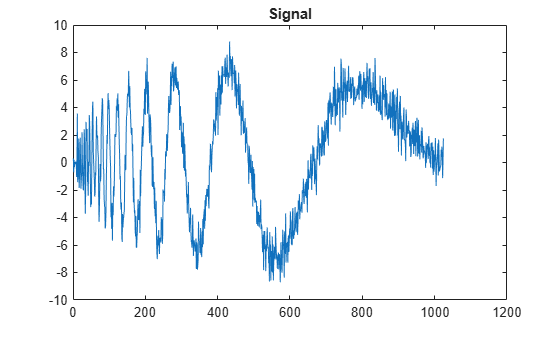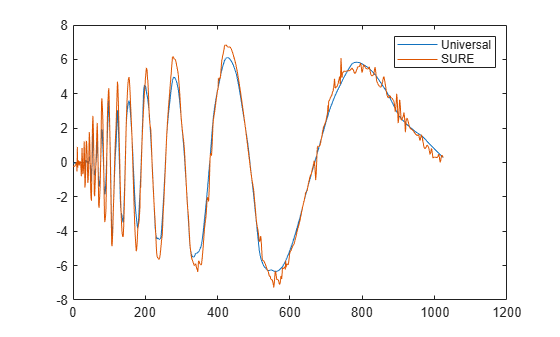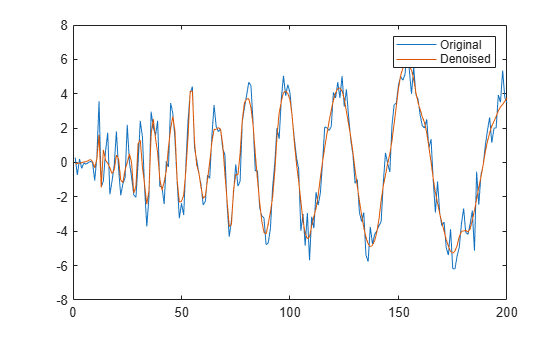# Denoise Signal Using Generated C Code

This example shows how to denoise a signal using C code generated from the `wdenoise`function. Two techniques are demonstrated. The first technique uses a wrapper function which calls `wdenoise`. The second technique generates C code directly from `wdenoise`. Depending on your workflow, one technique may be preferred over the other. For example, to streamline code generation for large MATLAB® code, you can use wrapper functions to modularize the code.

### Use Wrapper Function

Create a MATLAB function `denoiseSignal` that executes `wdenoise` and returns a denoised signal. The function takes two input arguments: a 1-D signal, and a denoising method. It passes these arguments to `wdenoise`. The function is included in the directory containing this example. The code for `denoiseSignal` follows.

```function out = denoiseSignal(input,denMthd) %#codegen out = wdenoise(input,'DenoisingMethod',denMthd); end ```

The `%#codegen` directive indicates that the function is intended for code generation.

Use `codegen` to generate a MEX function. Code generation defaults to MEX code generation when you do not specify a build target. By default, `codegen` names the generated MEX function `denoiseSignal_mex`. To allow the generation of MEX file, specify the properties (class, size, and complexity) of the two input parameters:

• `coder.typeof(0,[1 Inf])` specifies a row vector of arbitrary length containing real `double` values.

• `coder.typeof('c',[1 Inf])` specifies a character array of arbitrary length.

`codegen denoiseSignal -args {coder.typeof(0,[1 Inf]),coder.typeof('c',[1 Inf])}`

```load noisdopp plot(noisdopp) title('Signal')```Denoise the signal twice using `denoiseSignal_mex`. First, use the denoising method `UniversalThreshold`. Then, use the `SURE` method. Plot both results.

```dn = denoiseSignal_mex(noisdopp,'UniversalThreshold'); dn2 = denoiseSignal_mex(noisdopp,'SURE'); plot([dn' dn2']) legend('Universal','SURE')```Use the MATLAB function and MEX function to denoise the signal using the `Minimax` method. Confirm the results are equal.

```dnA = denoiseSignal_mex(noisdopp,'Minimax'); dnB = denoiseSignal(noisdopp,'Minimax'); max(abs(dnA-dnB))```
```ans = 6.2172e-15 ```

### Use Explicitly

You can also generate C code directly from `wdenoise`. Generate a MEX file that denoises a signal using the `db4` wavelet. The generated MEX file is called `wdenoise_mex`.

`codegen wdenoise -args {coder.typeof(0,[1 Inf]),coder.Constant('Wavelet'),coder.Constant('db4')}`

Denoise the original signal.

`dn3 = wdenoise_mex(noisdopp,'Wavelet','db4');`

To confirm the MEX file accepts variable length inputs, denoise the first 200 samples of the original signal.

```dn4 = wdenoise_mex(noisdopp(1:200),'Wavelet','db4'); plot([noisdopp(1:200)' dn4']) legend('Original','Denoised')```